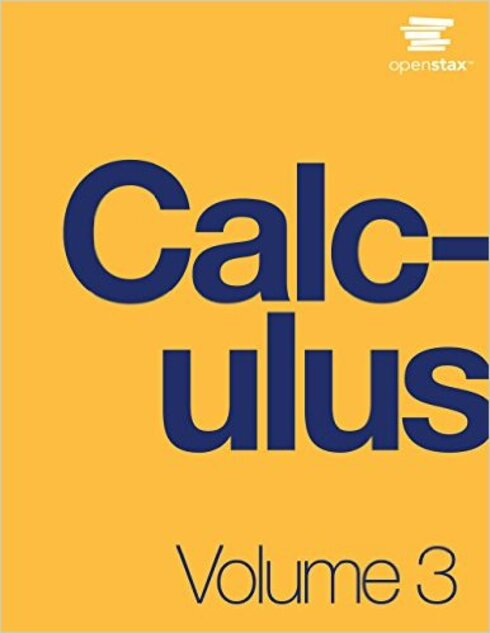# Calculus Volume 3

(2 reviews)Gilbert Strang, Massachusetts Institute of Technology

ISBN 13: 9781938168079

Publisher: OpenStax

Language: English

## Conditions of UseAttribution-NonCommercial-ShareAlike
CC BY-NC-SA

## Reviews

1. Parametric Equations and Polar Coordinates

• 1.1. Introduction
• 1.2. Parametric Equations
• 1.3. Calculus of Parametric Curves
• 1.4. Polar Coordinates
• 1.5. Area and Arc Length in Polar Coordinates
• 1.6. Conic Sections

2. Vectors in Space

• 2.1. Introduction
• 2.2. Vectors in the Plane
• 2.3. Vectors in Three Dimensions
• 2.4. The Dot Product
• 2.5. The Cross Product
• 2.6. Equations of Lines and Planes in Space
• 2.8. Cylindrical and Spherical Coordinates

3. Vector-Valued Functions

• 3.1. Introduction
• 3.2. Vector-Valued Functions and Space Curves
• 3.3. Calculus of Vector-Valued Functions
• 3.4. Arc Length and Curvature
• 3.5. Motion in Space

4. Differentiation of Functions of Several Variables

• 4.1. Introduction
• 4.2. Functions of Several Variables
• 4.3. Limits and Continuity
• 4.4. Partial Derivatives
• 4.5. Tangent Planes and Linear Approximations
• 4.6. The Chain Rule
• 4.7. Directional Derivatives and the Gradient
• 4.8. Maxima/Minima Problems
• 4.9. Lagrange Multipliers

5. Multiple Integration

• 5.1. Introduction
• 5.2. Double Integrals over Rectangular Regions
• 5.3. Double Integrals over General Regions
• 5.4. Double Integrals in Polar Coordinates
• 5.5. Triple Integrals
• 5.6. Triple Integrals in Cylindrical and Spherical Coordinates
• 5.7. Calculating Centers of Mass and Moments of Inertia
• 5.8. Change of Variables in Multiple Integrals

6. Vector Calculus

• 6.1. Introduction
• 6.2. Vector Fields
• 6.3. Line Integrals
• 6.4. Conservative Vector Fields
• 6.5. Green's Theorem
• 6.6. Divergence and Curl
• 6.7. Surface Integrals
• 6.8. Stokes' Theorem
• 6.9. The Divergence Theorem

7. Second-Order Differential Equations

• 7.1. Introduction
• 7.2. Second-Order Linear Equations
• 7.3. Nonhomogeneous Linear Equations
• 7.4. Applications
• 7.5. Series Solutions of Differential Equations

Table of IntegralsTable of DerivativesReview of Pre-Calculus

## Ancillary Material

• OpenStax

Calculus is designed for the typical two- or three-semester general calculus course, incorporating innovative features to enhance student learning. The book guides students through the core concepts of calculus and helps them understand how those concepts apply to their lives and the world around them. Due to the comprehensive nature of the material, we are offering the book in three volumes for flexibility and efficiency. Volume 3 covers parametric equations and polar coordinates, vectors, functions of several variables, multiple integration, and second-order differential equations.